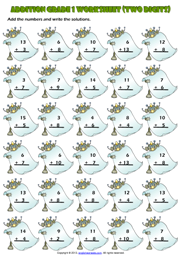Home Maths Main Page

# Addition Exercise Maths Worksheet With Two Digit Results

A fun addition exercise maths worksheet for grade 1 (first grade) students and kids with one digit and two digits numbers and robots theme. Add the numbers and write the solutions. (Vertical Addition Worksheet with two digit results for kids)